### Home > MC1 > Chapter 9 > Lesson 9.2.2 > Problem9-74

9-74.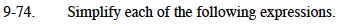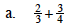Convert both terms to equivalent fractions with a denominator of 12.

$\left(\frac{2}{3}\right)\left(\frac{4}{4}\right)+\left(\frac{3}{4}\right)\left(\frac{3}{3}\right)=\frac{8}{12}+\frac{9}{12}$

$\frac{8}{12}+\frac{9}{12}=\frac{17}{12}$

$\frac{17}{12}=1\frac{5}{12}$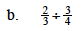Remember that dividing by a fraction is the same as multiplying by its reciprocal.
How could you rewrite this division problem as a multiplication problem?

$\left(\frac{2}{3}\right)\left(\frac{4}{3}\right)=\frac{8}{9}$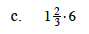Convert the mixed number to a fraction greater than one before multiplying.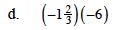Remember that the product of two negative numbers is positive.